• 1
•
•
•
•
•
•
•
1
Share

# GAUSS’S LAWS

Without Gauss Theorem study of electrostatic is incomplete. So it became necessary to know about gauss theorem. In this article we are going to discuss about gauss theorem in detail. So keep reading till end.

In electromagnetism, gauss’s law is also known as gauss flux’s theorem. It is a law which relates the distribution of electric charge to the resulting electric field. Gauss’s Law is mathematically very similar to the other laws of physics. Such as – gauss’s law for magnetism, gauss’s law for gravity.

However, gauss’s law can be expressed in such a way that it is very similar to the inverse square law. In physics there are many inverse square laws, such as inverse square coulomb’s law, inverse square Newton’s laws of gravity , inverse square magnetic coulomb’s law etc. Gauss’s law is essentially very equivalent or analogies to these inverse square laws.

There are two forms in which gauss’s law can be expressed. We can express this law mathematically using vector calculus in integral form and in differential form, both form are equivalent since they are related by the divergence theorem which are also called Gauss theorem.

1). Integral form – This form of gauss theorem is used when distribution of electric charge on any closed surface is continuous or symmetrical. Integral form of gauss law states that electric flux through any arbitrary surface is proportional to the total electric charge enclosed by the surface.

2). Differential form –  This form of gauss theorem is used when distribution of electric charge over a closed surface is discontinious or unsymmetrical. Differential form of gauss law states that the divergence of the electric field is proportional to the local density of charge.

This law was first formulated by Joseph-Louis Lagrange in 1773, and further followed by Carl Friedrich Gauss in 1813. It is one of the Maxwell’s four equation. And this law can be used to derive coulomb’s law or vice versa.

Now we are going to talk about each form of gauss’s law in detail one by one.

## INTEGRAL FORM

### GAUSS’S LAW : STATEMENT

The gauss theorem states that – The surface integral of the electric field intensity over a closed surface (called Gaussian surface) in free space is equal to the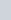times the net electric charge enclosed within the surface. Mathematically it is given as –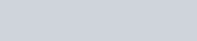Where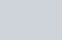is the algebraic sum of all the charge inside the closed surface. Hence, the total electric flux over a closed surface in vaccum istimes the total charge within the surface irrespective of how that charge is distributed.

### PROOF OF GAUSS’S THEOREM FOR SPHERICALLY SYMMETRIC SURFACETake a q charge and make a Gaussian surface of radius r. Now cut a small area element dS through which we will find electric flux. See fig. above : Electric flux through this small symmetrical area element(surface) dS is given as –Here,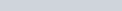, now the equation become: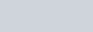Total electric flux through the spherical surface is given as-If electric charge is kept in a medium of dielectric constant k then-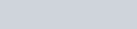Where,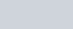And if there is no any charge enclosed in the Gaussian surface i.e q = 0 then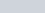## DIFFERENTIAL FORM

Using divergence theorem, gauss’s law can be expressed in differential form as follows: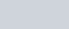where ∇ · E is the divergence of the electric field, ε0 is the electric constant also called permittivity of the free space, and ρ is the volumetric charge density (charge per unit volume).

### EQUIVALENCE OF INTEGRAL & DIFFERENTIAL FORM

Mathematically gauss law are equivalent in integral and differential form. We can proof this equivalency by using divergence theorem.

#### EQUIVALENCY PROOF

The integral form of Gauss’ law is: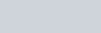And for any closed surface S containing charge Q. By the divergence theorem, this equation is equivalent to: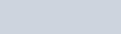And for any volume V containing charge Q. By the relation between charge and charge density, this equation is equivalent to: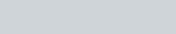for any volume V. In order for this equation to be simultaneously true for every possible volume V, it is necessary for the integrands to be equal everywhere. Therefore, this equation is equivalent to: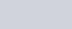Thus the integral and differential forms are equivalent.
Watch the video for more reference